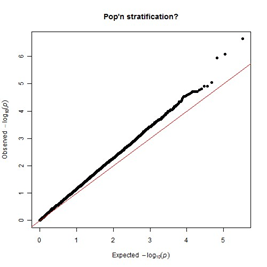Programming Assignment 1 – Association Statistics and Power COMSCI/HUMGEN 124/224 Solved

29.99 \$ 15.00 \$

Category:
Click Category Button to View Your Next Assignment | Homework

Description

This short programming assignment is designed to help you get an understanding for the basics of power and association computations from a computational persepctive. You can use any language for this project, though Python and R are recommended. You will need to submit your code along with your results file through CCLE.

Association Study at a Single SNP

The data for this programming assignment consists of a matrix of 2,000 individuals, 100,000 SNPs, and one column representing the individual’s status as a case or control. Unzip the file SNP_status.zip, and you’ll see a space-separated text file. Each column of the input represents a single SNP, and each row represents an individual. Each element in the matrix represents the number of copies of a single SNP an individual has.

To get started, you may want to look at the documentation for the Python module pandas read_csv function, or the documentation of R’s read.table function.

If you aren’t sure where that is, literally copy and paste the module and method you’re into Google.

That trick increases beginning computer programmer productivity by about 50 percent.

Part A – Computing Association Statistics

Your job will be to compute the association statistic, the non-centrality parameter, and the corresponding p-value for each SNP.

You should use the formulas that we have developed in class to compute association statistics. Use the statistic:

Once you have the association statistic, use the normal quantile function to compute p-values. Use a two-tailed test for significance; report the p-values as described in Part D.

Part B – Bonferroni Correction

Bonferroni correction is a way to maintain the ”family-wide error rate” (FWER) of a set of related tests. If we want perform m tests of significance level α on data that conform to the null hypothesis, we would expect to find tests passing that level of significance on average, under the null. When m is large To combat this, we make each test pass a more stringent threshold of.

For this assignment, use α = .05 for your two-tailed test. That is, a test is considered significant if and only if it is within the upper or lower 2.5% tails of the standard normal distribution.

Apply Bonferroni correction to your results from Part A and report the significantlyassociated SNPs as described in Part D..

Part C – Q-Q plots and Inflation Statistics (Optional for Undergrads, Required for Grads)

Recall that a p-value is the probability that an association statistic of a particular magnitude will appear in the data. Given that definition, we should expect that only 30% of the individual hypotheses that we test should have p-values should be stronger than .3. If we see more than 30% of our statistics with p-values stronger than .3, that indicates our statistical test may be detecting many false positives.

However, it is often the case that we see tests with significantly increased or decreased p-values with versus our expectation. One way that this increase or decrease is quantified is using a statistic called λgc, or the genomic control statistic.

Median empirical χ2 statistic λgc =

Median value of distribution

Using Python or R, find the median value of the chi-square (χ2) distribution with one degree of freedom.

You can compute (χ2)-distributed statistics by squaring the statistics you generated in Part A.

Compute λgc for your study, and report it as described in part D.

There are many tutorials on how to make a Q-Q plot online; if you are new to Python or R plotting, it is recommended that you adapt code from one of these to suit this purpose. If you’re stuck, Google ”QQ Plot R” or ”QQ Plot Python” and read the documentation and tutorials.

There are several ways to make a Q-Q plot; what they all have in common is that the x-value is the expected value of a statistic for a particular quantile, and the y-axis is the experimental value of the statistic at that quantile.Figure     1:             A             Q-Q         plot         of            p-values,               from http://www.gettinggeneticsdone.com/2011/04/ annotated-manhattan-plots-and-qq-plots.html

Some statistics you can use include your SA statistics from part A, which are distributed as a standard normal under the null hypothesis, the χ2 statistics you computed to compute λgc, which are distributed as a chi-square with one degree of freedom under the null, and the p-values, which should be distributed uniformly between 0 and 1 under the null. If you use p-values, it is generally more informative to show their negative log base 10. If you show the raw p-values, we will not be able to see the interesting very small p-values, since their numerical values will be clustered around 0.

Make an image of a Q-Q plot of your data and post it in the forums. Try to explain any trends you see.

Part D – Output Format

The most important part of this assignment is that you input and output data in the proper format. The first line should be your UID; it is recommended that you also put your UID in the title of your file, but it’s not required.

{SNPNAME}:{RAW-P-VALUE}

{SNPNAME}:{RAW-P-VALUE}

{SNPNAME}:{RAW-P-VALUE} …

</A>

<B>

{SIGNIFICANT-SNP1}

{SIGNIFICANT-SNP2} …

</B>

<C>

Lambda_gc:<Lambda Value>

</C>

If I were to submit an assignment, my output would look something like this:

UID:123456789 email:[email protected]

<A>

SNP0000:0.175

SNP0001:0.875

SNP0002:0.0003 …

</A>

<B>

SNP0002 …

</B>

<C>

Lambda_gc:1.872

</C>